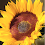## Wednesday, June 1, 2011

### Problem Solving: Fractions

Problem solving activities help students develop conceptual understanding of fractions as they use appropriate fraction models to think about and solve the different tasks.  These Mathwire open-ended assessments were designed to measure students' conceptual understanding of fractions.

Pattern Block Fraction Design requires students to fill a shape with pattern blocks to create a design that meets certain requirements.   Students must also write a fraction that describes the part of the total design represented by each different color pattern block.

Fraction Game simulates a Fraction War game but students must draw a representation of each fraction and explain who won, based on the drawings.  This assessment was designed for students in an Everyday Math program.  Students may draw any representation that helps them decide which fraction is larger and explain their thinking.

Mrs. Meatball challenges students to solve pizza fraction problems pictorially.  The problems are listed on one page, but students should use lots of room to draw pictures, label and explain how they solved each problem pictorially.  NOTE:   This task was originally designed for a teacher in-service class where participants were not allowed to use any previous fraction knowledge to solve the problems.  They were instructed to use only words, pictures and numbers to solve the problems and explain their thinking.  They were not allowed to use any fraction algorithms, etc.

1.Here i am telling you The Fastest Way To Solve Fractions(Addition) Through Vedic Math, TRIX FOR THE SAT

Take these fractions for example
1/2 +1/3
how do u add them fast
well first we need to cross multiply
1*3=3
1*2=2
u get the numbers three and two now simply take the to answers and do the following
3+2
____

3*2

5/6
there u go u get the answer and u can use this for any addition of fractions

--------------------
----
hope this helps
**NICK**

2.Thanks for the trick, Nick. In the future it would be helpful if your sample did not include repeat digits, in this case two 1s as numerators. This makes it a bit tougher to follow which digits you are adding and which you are multiplying.

I agree that the trick works, but clarity would impress those new to this method.